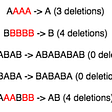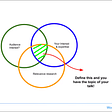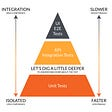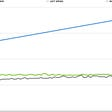# Drawing Book HackerRank Problem

Task :- Given N and P ,return minimum no of turns required.We can start from first page or last page

Brute Force solution :- Create a counter, start from 1 and keep on incrementing 2 till we get P.Create another counter, start from N,keep on decrementing 2 till we get P. Return the minimum of these two counts.

Brute Force code :-

Efficient Logic :- Since we have common increment or decrement we can use the concept of Arithmetic Progression where we are given the start and target with common difference.

For the first case when we start from 1, the answer simply becomes floor value of p/2.

For the second case when we start from n, there are two conditions:

• If n is odd, the answer becomes floor value of (n-p)/2
• If n is even, the answer becomes floor value of (n-p+1)/2.

The +1 is because the last page number n is on the left side and maps it correctly.

So we return the minimum of these two values.

Efficient Code:

--

--

--

Love podcasts or audiobooks? Learn on the go with our new app.## More from Medium